﻿ Optimal Design and Comparative Analysis of FOPID Controller for Three Area LFC Tuned Using EHO and GCMBO AlgorithmsPublications are Open
Access in this journal
Article Versions
Export Article
• Normal Style
• MLA Style
• APA Style
• Chicago Style
Research Article
Open Access Peer-reviewed

### Optimal Design and Comparative Analysis of FOPID Controller for Three Area LFC Tuned Using EHO and GCMBO Algorithms

D. K. Sambariya, Omesh Nagar
International Transaction of Electrical and Computer Engineers System. 2018, 5(1), 6-14. DOI: 10.12691/iteces-5-1-2
Published online: July 27, 2018

### Abstract

In this paper, signified a novel method to strategy of FOPID controller for LFC of a three area generating units. In this system, LFC of generating units of power plants has been used in frequency control due to having the better responses. The EHO and GCMBO are also considered using the parameters of FOPID controllers as a minimization of error in this optimization technique. The Integral Squared Error (ISE) is the objective function for optimum strategy of FOPID parameters of the EHO and GCMBO technique. Its gives the better dynamic response as compared the other FOPID controller parameters. The modal of generating units power plant with FOPID controller is simulated in MATLAB/SIMULINK platform. In this study, simulation explorations on a three-area LFC with different generating units. The effectiveness of the proposed methodology is better in terms of settling time, rise time, and peak time. The perturbation in load, change the system frequency which further increase the Undershoot of the system here, the undershoot of the system is decrease for EHO and GCMBO technique is used to tuned the parametric value of FOPID controller.

### 1. Introduction

Mostly interconnected areas in electric PS system LFC have a specific role. The LFC is attempt to uphold the system frequency of each area and inter area tie-line power with admissible limits to deal with several load demand and system disturbance. Therefore, LFC crisis received more attention in literature for power generating unit on fascinating topic. Most trust worthy and frugal of PS needs power balance between total load demand of power generation and associated system losses. The main objective of LFC in PS is to constitute system frequency during sudden load disturbance and variations. Load demand value varies randomly as it is not constant fixed and predictable. The value various due to tremendous development and discover in technology and industries. LFC in PS attend large attention currently and it is one of the most challenging problem in control engineering. Das, 2015 1 have proposed the work of Linear Quadratic Regulator based technique. Arunachalam, 2016 have reported the Fuzzy Logic Controllers and the Z-N is a tuning method. In the perspective of development in size of PS with economical change, power supply with constant frequency become very essential, which challenges present traditional methods 2, 3.

Sambariya et. al., 2017 4, 5 have presented the EHO technique for the application of LFC and tuning the PID parameters. In modernistic time, kinetic energy of water and the thermal energy of fossil fuels and nuclear fission are main source of electrical energy. This energy is converted by prime movers into mechanical energy and further synchronous generator into electrical energy. Governing system for controlling power and frequency provided by prime mover this operation coined by the term of LFC. Deo et al., 2016 6 have presented the manual optimization technique, for drive the FOPID controller. Shayanfar, 2016 7 have proposed the work of SSO technique-based tuning of the FOPID parameters. The main aim of electrical power system is to control the balance between total power generation with total load demand and associated system losses 8. Then regulating the system frequency and tie-line power exchange. It is mandatory to regulate generation loads depending on optimal frequency value through a secondary controller to assume the quality of power supply. This strategy known as LFC 9. Taher, 2014 2 have proposed the work of Imperialist Competitive Algorithm (ICA) and the FOPID parameters are tuned by the ICA technique. It is the most control problems in operation and design of PS which help to keep frequency unchanged by load the last but not least objectives of this system is to make right and correct value of interchanged power between control areas, to maintain every unit generation at most economical value the secondary objective is to ensure the non-violation of operating limits.

Bayati, 2015 8 have reported the LFC system for the interrelated PS and also used the integer order PID controller. FOPID parameters are tuned by the GA technique. Pan, 2013 10 have proposed the LFC system for the interrelated PS and used in the Non-Dominated Sorting Genetic Algorithm. For sufficient supply and reliable electrical power of good quality. LFC is very important in design, operation and control of large scale electrical power system that having interconnected control on region for sufficient supply and reliable electrical power of good quality. Mismatches in frequency and scheduled tie-line power flows between areas is used to restore and maintain by LFC. Chiranjeevi, 2014 11 have reported the LFC system for interconnected PS and FOPID parameters are tuned by the PSO technique. Shayeghi, 2016 12 have proposed the work of SSO technique-based tuning of the FOPID parameters. Gupta, 2015 13 have reported the Time- Response Specification Based Fitness and FOPID parameters are tuned by the PSO Algorithm. In case of abnormal condition and area load changes such as outage of generation and varying system parameters. the main objective of the LFC system is a zero-steady state error 14, 15.

This paper also designated the FOPID controller parameters are tuned by the EHO technique. This controller demonstrations toughness at the modification in system parameters. the proposed parameters are improved the concerts of the frequency unconventionality and tie-line power. The proposed controller technique according to EHO improved the dynamic response of change in frequency. EHO gives the better response as compared to Imperialist competitive algorithm.

This paper is described in five steps. The II step are also designated problem formulation with introduction to the measured the PS. The basics of FOPID controller objective functions. The III step are also representing the operational part and EHO. The IV step show the comparisons and simulation results. V step are presented the conclusion with references.

### 2. Problem Formulation

2.1. System Description

The electric power is generating the steam extended on the turbine in thermal plant Figure 1 2. The operation and controlling are also different in each all power plants. The TF of the thermal-hydro power plant as paralleled in Eq. 1-Eq. 6 5.

2.1.1. Speed Governor

The Eq. 1 5 define the speed governor typical of the LFC. Speed governor of the constant time is specified by TG and value of the parameters TG1=0.1, TG2, 3=0.2.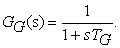(1)

2.1.2. Non-Reheat Turbine

The Eq. 2 5 define the TF of the turbine model. Turbine of the constant time is specified by TT. The value of the parameters Tch1,2=0.3.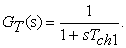(2)
• Figure 1. Three-area power system with different generating units

2.1.3. Re-Heater

In this system re-heater is increased the efficiency of a power plant. The boiler of the partial expansion is returned steam working of re-heating turbines. Eq. 3 5 represent the TF of re-heater model. The Trh and Tch are the low pressure reheat and non-reheat turbine time constants, Fhp are the high-pressure stage. Value of the parameters Trh=7, Tch1,2=0.3 and Fhp=0.3.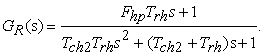(3)

2.1.4. Hydraulic Amplifier and Turbines

The Eq. 4 – Eq. 6 5 define the TF of hydraulic amplifier and hydraulic turbine model. Stage I the T1 and T2 are the hydro governor of constant time and stage II the hydro turbine individually Tw denoted the hydro turbine of the constant time.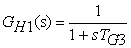(4)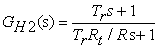(5)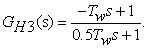(6)
2.2. Controllers
2.2.1. Fractional Calculus

Fractional operator is denoted by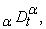where the Fractional operative is a simplification of integral & differential operators can be described with their equation of the fractional order controller (FOC’s). That is as: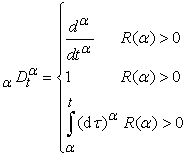(7)

Raimann-liouville in Eq. 8 16.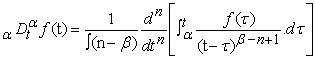(8)

Grunwald-Letinikov in Eq. 9 16.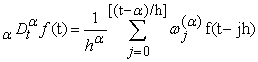(9)

Caputo as in Eq. 10 16.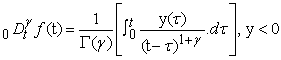(10)

This paper analysed first method to estimate of integer order transfer functions. FOPID have five unceasing estimates that are accessible such as crone, Carlson, Matsudo et. al. estimates the high frequency sustained segment estimate & the low frequency sustained segment estimate [ 17].

2.2.2. PID Controller

PID controller have several applications by using flexible feedback controller. It has an three mode of parameters these are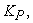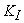and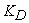, where its parameter value depends on mainly three actions (P), (I) and (D), P depends on the present error, I on past errors and D for future errors shows in Figure 2 16. Industrial control system is also measured by the PID controller because the increase and decrease the dynamic response with decay in the steady state error as in Eq. 11 and Eq. 12 18.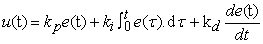(11)

Where, e(t) is error of the system,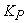,andis proportional, integral and derivative gain and u(t) is the controller of output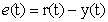(12)

Where, y(t)= output plant, r(t)=input reference signal

The transfer function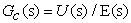of the PID controller expressed as in Eq. 13 19.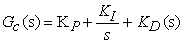(13)
• Figure 2. PID Controller

2.2.3. FOPID Controller

One of the most appropriate controller is the PID controller that the main applications of the industrial. FOPID controller have two extra parameter such as derivative (λ) and integrator (μ), This controller is called the PIλDμ controller. Hence, the difference between the FOPID and PID controller is the instruction of calculus operatives that make more degree of liberty and flexibility in strategy in Eq. 14 16.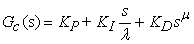(14)

Where, the KP, KI and KD are proportional, integral and differential gains and the μ and λ are fractional order of derivative and integrator shows in Figure 3 6. The shown in figure of FOPID controller is given below.

• Figure 3. FOPID controller

2.2.4. Objective Function

The objective function is signified the integral of squared error. The process to control the unidentified parameters of the FOPID controller (KP, KI, KD, λ and μ) are also the considered that the optimization problematic. This problem is measured the error signal in ISE Eq. 15 4. These parameters are also upper and lower bounds with both of the EHO and GCMBO technique.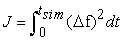(15)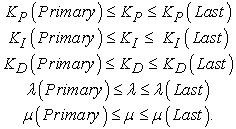(16)

### 3. Optimization Technique

3.1. Elephant Herding Optimization Technique

Due to growing complication, the actual-world problematic is difficult that it cannot be resolved by consuming this procedure. Some of the procedure based on the natural phenomenon cannot give accurate responses. In this time period researcher’s studies on several kinds of meta heuristics algorithm such as EHO. So, it can be easily applied to solved numeral of problematic in actual world.

3.1.1. Herding Behaviour

In this technique, the elephant is the largest animal which originate in group of mammals on the planet. Elephants has behavioural system, further size and domestic atmosphere. Eelephant lives in composite structure such as female elephant with her calves linked other female elephants. This set are also known as clan. A clan of a group is represented as in Figure 6. An elephant assembly contains the number of clans. A clan contains of lowest of two dozen elephants. The head of the clan are also known as the matriarch which control all concentric loops. When growing up male elephant lives alone in group and female elephant similar to lives in combined family. the male elephant mixed with few other adult male elephants in small group leaves the clan in Figure 6. They live in connection with their clan through lowest frequency of ambiances. The populace of all elephants as shown in Figure 4 [ 20].

• Figure 4. Populace of elephant

EHO is separated in two operatives. The operation of updating the clan and separating used to explain the entire optimization algorithm problem.

• Figure 5. Clan of generation
3.1.2. Procedure of Optimization

The following steps may be use to solve the selected problem by expiations herding behaviour.

It is supposed that the overall populace of elephants is separated into two parts such as clans. These clans have a certain sum of an elephants.

It is also supposed that the poorest performance of the male elephant will be left the assembly. It lives single on an extraordinary detachment from the elephant assembly.

All elephants leader is matriarch and it lives in a clan.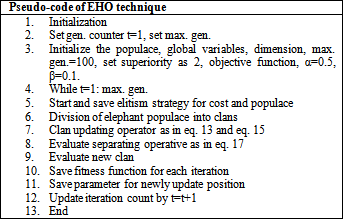• Figure 6. Clan updating operator
• Figure 7. Clan separating operatives

3.1.3. Clan Modernized Operator

Matriarch is controlled the clan; CP is the overall number of clans and q shows the number of elephants. The existing situation of all elephants are modernized as in Eq. 17 5.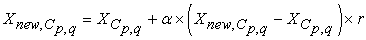(17)

Where, Xnew,Cp,q and XCp,q are the old position and new update for elephant q in clan Cp correspondingly. α is a scale factor and Xnew,Cp describe the best position in matriarch. Its clan cannot be updated in Eq. (18) 4.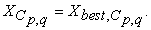(18)
• Figure 8. Flowchart of EHO

The matriarch is modernized by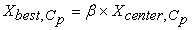(19)

Where, β=[0,1], β is a factor.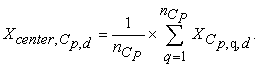(20)

The clan updating operative of EHO technique can be formed as shown in Figure 8.

3.1.4. Clan Separating Operator

Let us consider the elephant separate with the poorest performance will implement the separating operative at each group in Eq. 21 20.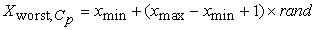(21)

Where, xmax and xmin signifies the max. and min. position of the elephants. The stochastic circulation operative, “rand” is a random numeral.

3.2. Greedy Strategy with Improved Crossover Operator-Based Monarch Butterfly Optimization (GCMBO) Algorithm
3.2.1. Monarch Butterfly Optimization

The migration of monarch butterfly is simplified some rules.

The whole population compared the Land 1 and Land 2 in Monarch butterflies.

The butterflies are also so generated the Land 1 and Land 2 of each offspring.

The size of the butterfly population keeps unmovable during the optimization procedure. The butterfly populace has an unmovable optimization procedure.

The butterfly adjusting and migration operator cannot be updated in the fittest number of monarch butterfly.

3.2.1.1. Migration Operator

In monarch butterflies the Land 1 and 2 are also measured ceil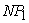sub populace 1 and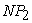sub populace, individually.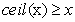NP is denoted the populace size. P is the Land 1 of Monarch butterfly. The migration operator given in Eq. 22 21.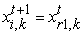(22)

Where,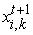is the element of kth of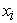at generation t+1. Correspondingly, the current generation numeral denotes the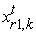.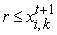is generated by Eq. 23 22.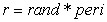(23)

Where, migration operator is denoted peri is set to basic MBO technique.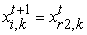(24)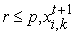is generated by Eq. 24,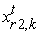is described the element of Kth and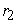is a butterfly selected from sub populace.

If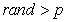, it can be updated as in Eq. 25.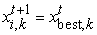(25)

Where,is the element of kth ofat generation t+1. Correspondingly,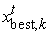denote the fittest butterfly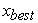. Againupdated as in Eq. 26.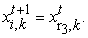(26)

Under this disorder,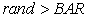updated form as follows in Eq. 27.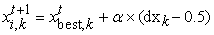(27)

Where, adjusting rate of BAR, j is a walk stage, now calculate by Levy flight given in Eq. 28.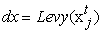(28)

Weighting factor as shows in Eq. 29 23.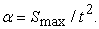(29)

3.2.2. GCMBO Algorithm

MBO is a new vigorous metaheuristic technique, and it has established its performance on standard development. However, MBO cannot reach the optimal performance on average. We have a used two policies unified the basic MBO technique with the main aim of improve the performance of MBO, the processor of GCMBO technique are introduced 21.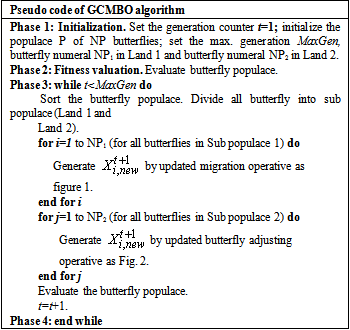3.2.2.1. Updating Migration Operator

By subsequent the conventions as presented previous, the numeral of butterflies in Land 1 and 2 are described as measured ceilsub populace 1 andsub populace, individually.NP is denoted the populace size separately. In the basic MBO technique newly-created butterfly are recognized and allowed to be permitted the next stage. GCMBO greedy strategy confirm that the butterfly individuals have some better performances are recognized to passage the succeeding stage as shows in Eq. 30 23.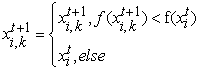(30)

Where,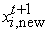is the next stage of butterfly,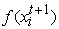or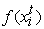butterfly fitness.

All the butterfly follows the self-adaptive operator and greedy strategy are unified the optimization procedure and generated the butterfly individually. Butterfly adjusting operator shown in Eq. 31 22.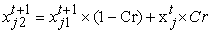(31)

Where,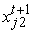is created the butterfly independently by usingand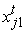and crossover rate is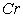. Self-adaptive strategy is presented as follows in Eq. 32: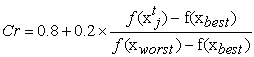(32)

Where,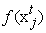is butterfly fitness j is Sub Populace 2;and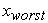is presented the entire butterfly populace. The new butterfly individual is determined by using greedy strategy shows in Eq. 33 24.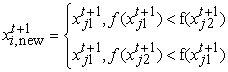(33)

Where,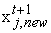is newly-generated butterfly for the next stage.

### 4. Simulation and Discussion

In simulation study, we simulate the three-area LFC with FOPID controller using EHO and GCMBO technique. The parameter of the TF used in the model is shown in Table 1. The parameters of three area power system is shown in Table 2. optimization process to tune the FOPID parameters is considered with objective function as in Eq. 15. The plot of the fitness function during the optimization in terms of iteration count is shown in Figure 9 and Figure 10. The optimal parameters using EHO and GCMBO and in literature are shown in Table 2. The step response comparison is included in Table 3 and Table 4.

• Figure 9. Fitness function of EHO
• Figure 10. Fitness function of GCMBO
• Figure 11. Change in the frequency of area 1 for step increase indemand of area 1
• Figure 12. Change in the frequency of area 2 for step increase indemand of area 2
• Figure 13. Change in the frequency of area 3 for step increase in demand of area 3
• Figure 14. Change in Ptie1
• Figure 15. Change in Ptie2

The model of three-area generating units of LFC control using FOPID controller tuned by EHO and GCMBO technique is settled in MATAB / SIMULINK platform. Now, we simulate the three-area plant with FOPID controller using EHO and GCMBO. We compared the proposed FOPID controller with other controller of taher in result of 1% step load perturbations or are also applied on the power demand of generating units. The generating power plants are compared the responses of the frequency deviation. The controller is demonstrated the toughness of the system to variation in the operational state and system parameters diverse within the limit +25% to -25%. The settling time and undershoot Table 3 of frequency deviation satisfactory ranges. Hence so that the proposed controller is robust, the parameter changes within the range of +25% to -25%.

4.1. Performance Comparison

Three-area generating units is measured of the FOPID controller for the load frequency control problems. The FOPID controller parameters of the EHO technique gives the less undershoot of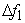= -0.091,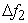=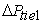= -0.098,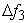=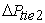= -0.103, and the parameters of GCMBO technique gives the less undershoot of= -0.093,== -0.097,== -0.109, where the undershoot of taher are= --0.097,== -0.104,== -0.111. The proposed undershoot value better as compared to taher’s parameters values. The comparison of frequency unconventionality on the source of undershoot and settling time tabulated in Table 3 and Table 4.

### 5. Conclusion

In this article, the FOPID controller for an LFC is established forward in a three-area power system using EHO and GCMBO technique. The parameters of the FOPID controller is tuned by the EHO and GCMBO technique for solve the frequency problem to minimize diminished the variation of frequency in three-area generating units. The efficiency of the proposed controller is analysed by the simulation results. It’s have a better dynamic response as compared the taher’s parameters. The parameters of the EHO technique based FOPID controller are vital not to be variation even if the parameters are also extensive changed in loading condition. The simulation results of the proposed parameters have better results as compared the taher’s parameters. The undershoot is reduced by the proposed FOPID controller tuned by the EHO and GCMBO technique as associated to the controller.

### References

Published with license by Science and Education Publishing, Copyright © 2018 D. K. Sambariya and Omesh NagarThis work is licensed under a Creative Commons Attribution 4.0 International License. To view a copy of this license, visit http://creativecommons.org/licenses/by/4.0/